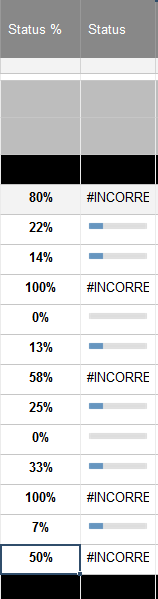# Nested IF Error - Incorrect Argument

Hi all,

Longtime reader, first-time poster...having a struggle day with the brain and some trouble with a nested if shooting back the INCORRECT ARGUMENT error.

Trying to code a 5-Part Progress Bar column with ranges based on a Status % column for a Project Tracker.

Here is what I have:

=IF([Status %]88 = 0, "Empty", IF([Status %]88 < 0.25, "Quarter", IF([Status %]88 = 0.25, "Quarter", IF([Status %]88 < 0.35, "Quarter", IF([Status %]88 < 0.5, "Half", IF([Status %]88, <0.65, "Half", IF([Status %]88 < 0.75, "Three Quarter", IF([Status %]88 < 1, "Three Quarter", IF([Status %]88 = 1, "Full")))))))))

Any help is greatly appreciated!!

• Added Screenshot, note formula stops after the Status79 <0.35 rule.• IF([Status %]88, <0.65   << The comma after [Status %]88 is wrong

Greetings

## Help Article Resources

Want to practice working with formulas directly in Smartsheet?

Check out the Formula Handbook template!